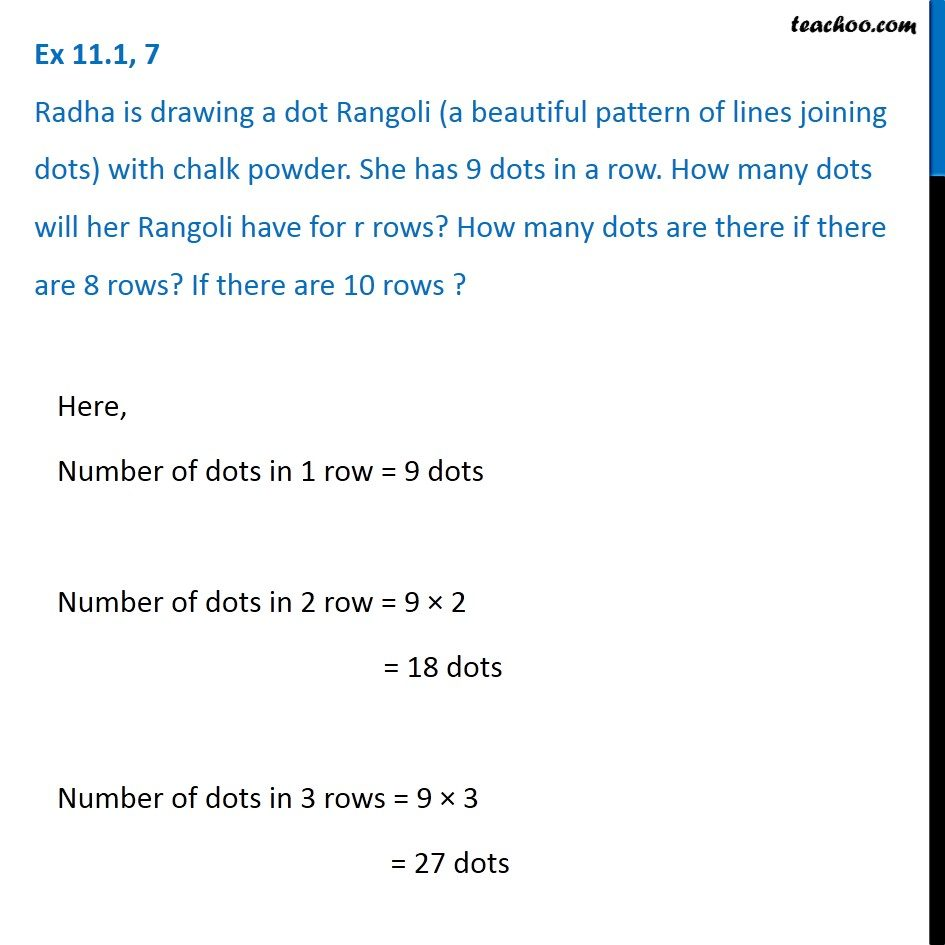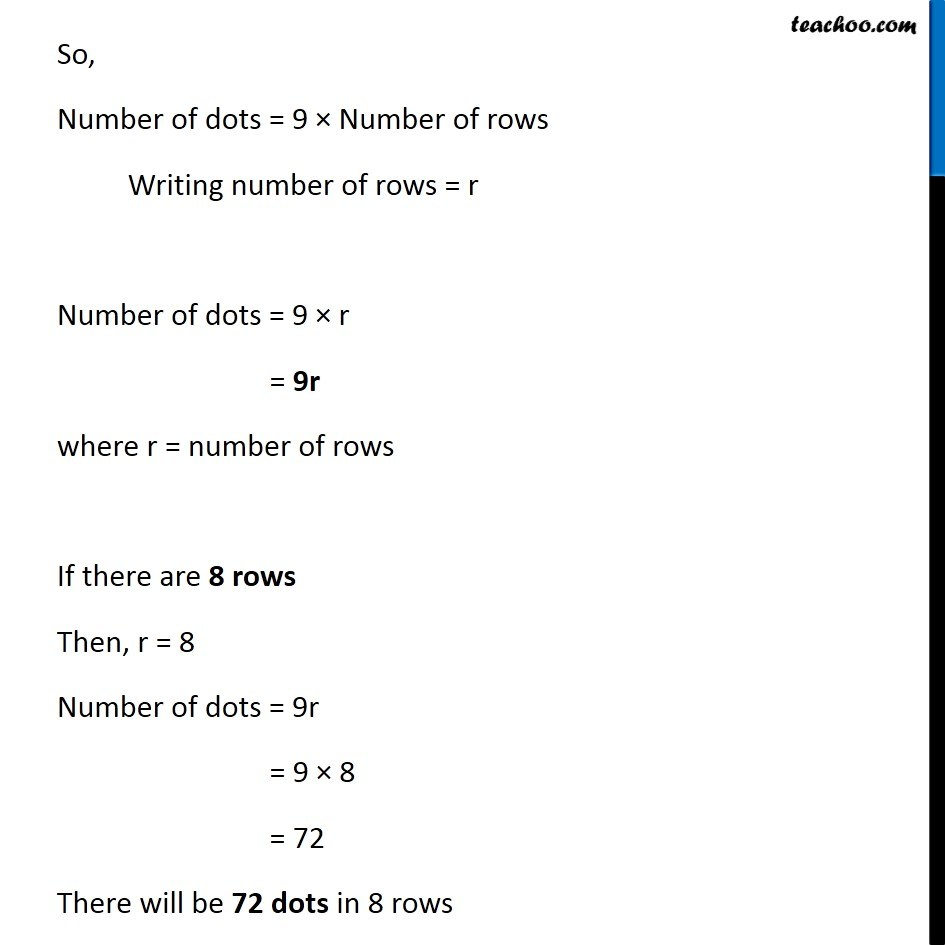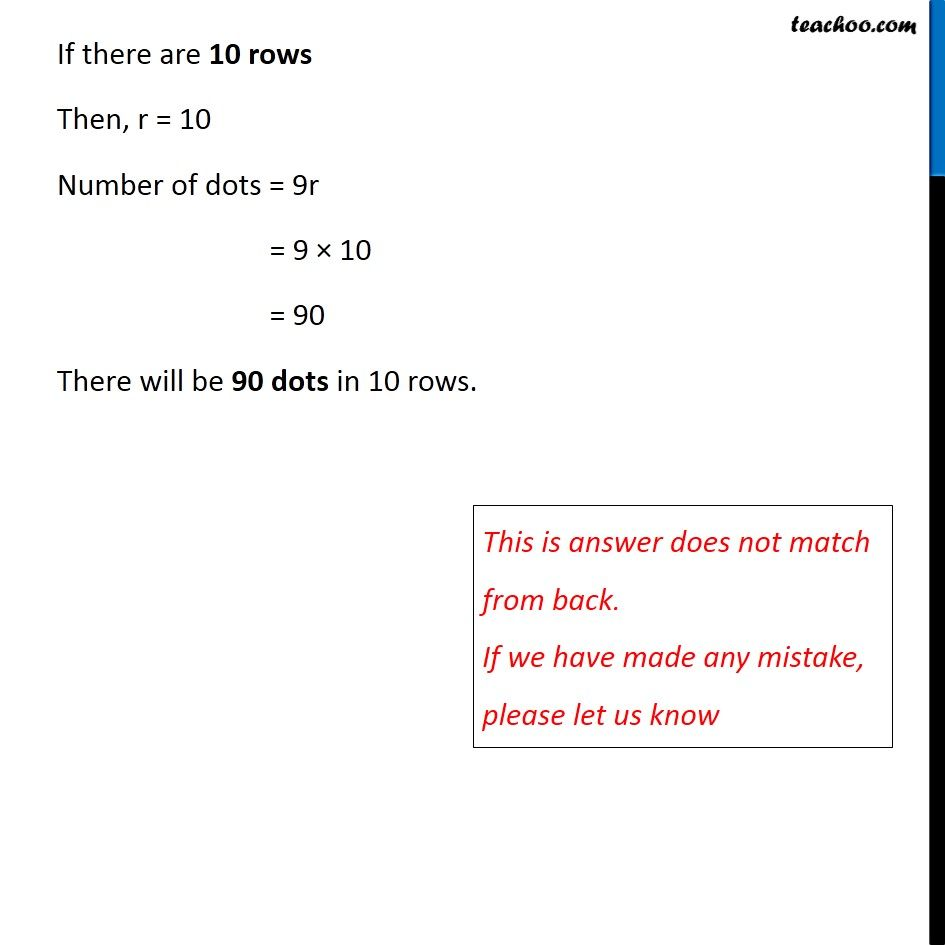Ex 11.1

Chapter 11 Class 6 Algebra
Serial order wiseLearn in your speed, with individual attention - Teachoo Maths 1-on-1 Class

### Transcript

Ex 11.1, 7 Radha is drawing a dot Rangoli (a beautiful pattern of lines joining dots) with chalk powder. She has 9 dots in a row. How many dots will her Rangoli have for r rows? How many dots are there if there are 8 rows? If there are 10 rows ? Here, Number of dots in 1 row = 9 dots Number of dots in 2 row = 9 × 2 = 18 dots Number of dots in 3 rows = 9 × 3 = 27 dots So, Number of dots = 9 × Number of rows Writing number of rows = r Number of dots = 9 × r = 9r where r = number of rows If there are 8 rows Then, r = 8 Number of dots = 9r = 9 × 8 = 72 There will be 72 dots in 8 rows If there are 10 rows Then, r = 10 Number of dots = 9r = 9 × 10 = 90 There will be 90 dots in 10 rows. This is answer does not match from back. If we have made any mistake, please let us know